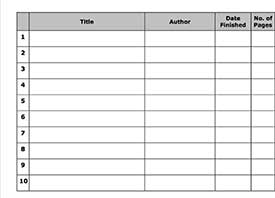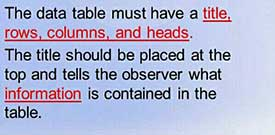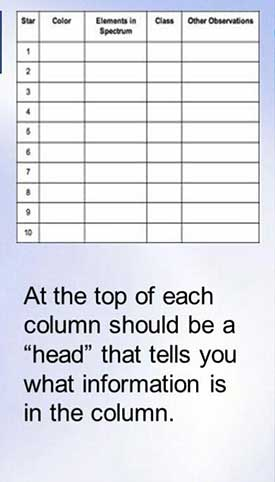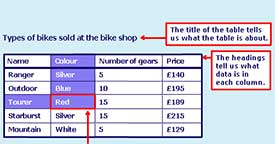Tables, Graphs, Mean  by  Rose Oduor

6

What is a data table in mathematics?It is where collected data is recorded in an organized fashion.

What must a data table have?What should the top of each column of a data table have?What does the title and heading tell us in a data?Identify how one can find out the colour of Tourer bike in the table below1 . The table below shows rainfall of Moyale in a certain year. Use it to answer the questions that follows

 Months J F M A M J J A S O N D Rainfall in mm 50 25 75 200 100 10 25 50 25 100 125 75

(a) Which month had the highest rainfall?

(b) Which was the driest month?

(c) What was the total annual rainfall?

1a. April

1b. June

1c. 860mm

2.The table below shows cars and buses imports of a country from 1999 to 2002. Use it to answer the questions below.

 YEAR CAR BUSES 1999 3000 1000 2000 3500 1500 2001 3000 2000 2002 5000 3000

(a) What was the total number of cars and buses imported in 1999?

(b) What was the total number of cars imported in 2000 and 2001?

(c) How many more buses were imported in 2002 than in 2001?

6

2

a. 4000

b. 6500

c. 1000

3. The table below shows the number of pupils absent in a class of 36 during one week

 Monday Tuesday Wednesday Thursday Friday Boys 5 2 1 2 4 Girls 3 2 2 3 1

(a) What was the total number of pupils absent during the week?

(b) What was the total number of pupils present during the week?

(c) On what day was the number of pupils present smallest?

(d) On what day was the number of pupils present highest?

3

a. 25

b. 11

c. Monday

d. Wednesday

4. The table below represents the mass of 19 pupils in a class

 Mass In Kilograms 25 27 30 35 Number of Pupils 4 7 5 3

a) How many pupils have the least mass?

(a) How many pupils have the highest mass?

(c) Which mass has most pupils?

4

a. 4

b. 3

c. Mass of 27kg